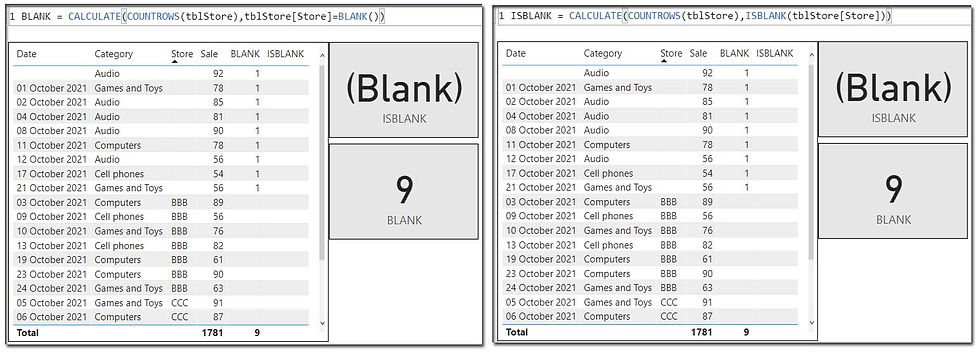top of page
Search
• Marek Vavrovic

# Information functions, part two

## ISBLANK

Checks whether a value is blank, and returns TRUE or FALSE.

ISBLANK(<value>)

return type : Boolean

Example 1

I want to return a UNICHAR() function in case, that there is a blank value in the sale column.Example 2

ISBLANK() vs BLANK()

BLANK() -- Returns blanks and empty string. Works with categorical and numerical data.

ISBLANK() -- Returns only blanks in the numeric data.

I want to calculate the number of missing stores in the tblStore[Store] column. These are the categorical data.

BLANK() function can works with the categorical data and returned a correct number of empty strings in the [Store] column.

ISBLANK() function does not work with empty string, returned (Blank)## ISEMPTY

Checks if a table is empty, contains any row.

return value: TRUE if the table is empty (has no rows)## ISERROR

I wrote a measure, that designedly generates an error, if used in a table or somewhere. This calculation is unprotected against any error messages.ISERROR is able to detect if its argument produces an error...ISERROR vs IFERROR

IFERROR evaluates an expression and returns a specified value if the expression returns an error; otherwise returns the value of the expression itself.note:

The IFERROR function is a faster implementation of a semantically equivalent IF( ISERROR () pattern.

IF ( ISERROR ( A ), B, A ) = IFERROR ( A, B )

In this case would be more natural to use the DIVIDE function, which contains an error handling logic in form of an alternate result.

DIVIDE(<numerator>, <denominator> [,<alternateresult>])